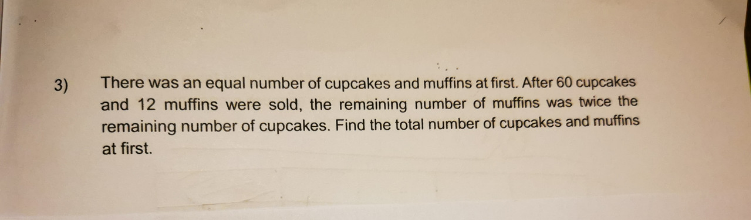# QuestionI’m pretty bad at models, but let me try…

C <——-60 —>
M M <—12—>

1 U + 60 = 2 U + 12

1 U = 60 – 12 = 48

So, at first, number of cupcakes = 48 + 60 = 108
number of muffins = 48 * 2 + 12 = 108
Total number of cupcakes and muffins = 108 + 108 = 216 #